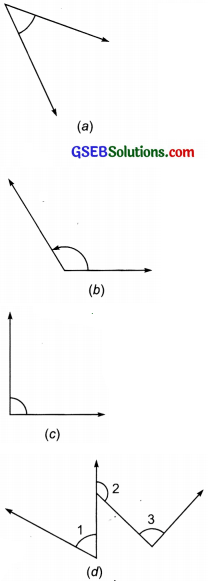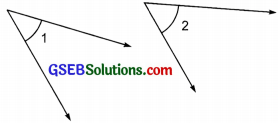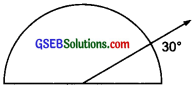# GSEB Solutions Class 6 Maths Chapter 5 Understanding Elementary Shapes Ex 5.4

Gujarat Board GSEB Textbook Solutions Class 6 Maths Chapter 5 Understanding Elementary Shapes Ex 5.4 Textbook Questions and Answers.

## Gujarat Board Textbook Solutions Class 6 Maths Chapter 5 Understanding Elementary Shapes Ex 5.4

Question 1.
What is the measure of
(i) a right angle? and
(ii) a straight angle?
Solution:
(i) The measure of a right angle = 90°
(ii) The measure of a straight angle = 180°

Question 2.
Say true or false:
(a) The measure of an acute angle < 90°
(b) The measure of an obtuse angle < 180° (c) The measure of a reflex angle > 180°
(d) The measure of one complete revolution = 360°.
(e) If m$$\angle A$$ = 530° and m$$\angle B$$ = 35°, then m$$\angle A$$ > m$$\angle B$$.
Solution:
(a) True
(b) False
(c) True
(d) True
(e) TrueQuestion 3.
Write down the measures of
(a) some acute angles and
(b) some obtuse angles.
(Give at least two examples of each)
Solution:
(a) 63° and 72° are acute angles.
(b) 120° and 100° are obtuse angles.

Question 4.
Measure the angles given below using the protractor and write down the measure.Solution:
(a) 45°
(b) 125°
(c) 90°Question 5.
Which angle has a large measure? First estimate and then measure.Measure of angle A =
Measure of angle B =

Solution:  Here,
Measure of angle A = 40°
Measure of angle B = 65°
Thus. $$\angle B$$ > $$\angle A$$

Question 6.
From these two angles which has larger measure? Estimate and then confirm by measuring them.Solution:
The opening of angle 2 seems to be more than angle 1.
Now, measure of angle 1 = 45°
and measure of angle 2 = 60°
Thus, the angle 2 has greater measure.Question 7.
Fill in the blanks with acute, obtuse, right or straight:
(a) An angle whose measure is less than that of a right angle is …………………..
(h) An angle whose measure is greater tizan that of a right angle is …………………..
(c) An angle whose measure is the sum of tile measures of two right angles is …………………..
(d) When the sum of’ the measures of two angles is that oía right angle, then each one of them is …………………..
(e) When the sum of the measures of two angles is that of a straight angle and f one of them is acute then the other should be ……………………
Solution:
(a) acute angle.
(b) obtuse angle.
(c) straight angle.
(d) acute angle.
(e) obtuse angle.

Question 8.
Find the measure of the angle shown in each figwe. (First estimate with your eyes and then find the actual measure with a protractor)Solution:
(i) Measure of the angle = 40°
(ii) Measure of the angle = 130°
(iii) Measure of the angle = 65°
(iv) Measure of the angle = 135°Question 9.
Find the angle measure between the hands of the clock in each figure:Solution:
The measure of the angle between the hands of a clock at:
(i) 9 : 00 a.m. is 90°
(ii) 1 : 00 p.m. is 30°
(iii) 6 : 00 p.m. is 180°

Question 10.
Investigate in the given figure, the angle measures 30°. Look at the same figure through a magnifying glass. Does the angle becomes larger ? Does the size of the angle change ?Solution:
No. Looking through a magnifying glass does not make the angle larger. No, the size of the angle does not change.Question 11.
Measure and classify each angle:Solution:
On measuring these angles, we have:
$$\angle AOB$$ = 40° $$\angle AOC$$ = 125°
$$\angle BOC$$ = 850 $$\angle DOC$$ = 950
$$\angle DOA$$ = 1400 and $$\angle DOB$$ = 180°
Thus, we can complete the given table in the following manner: